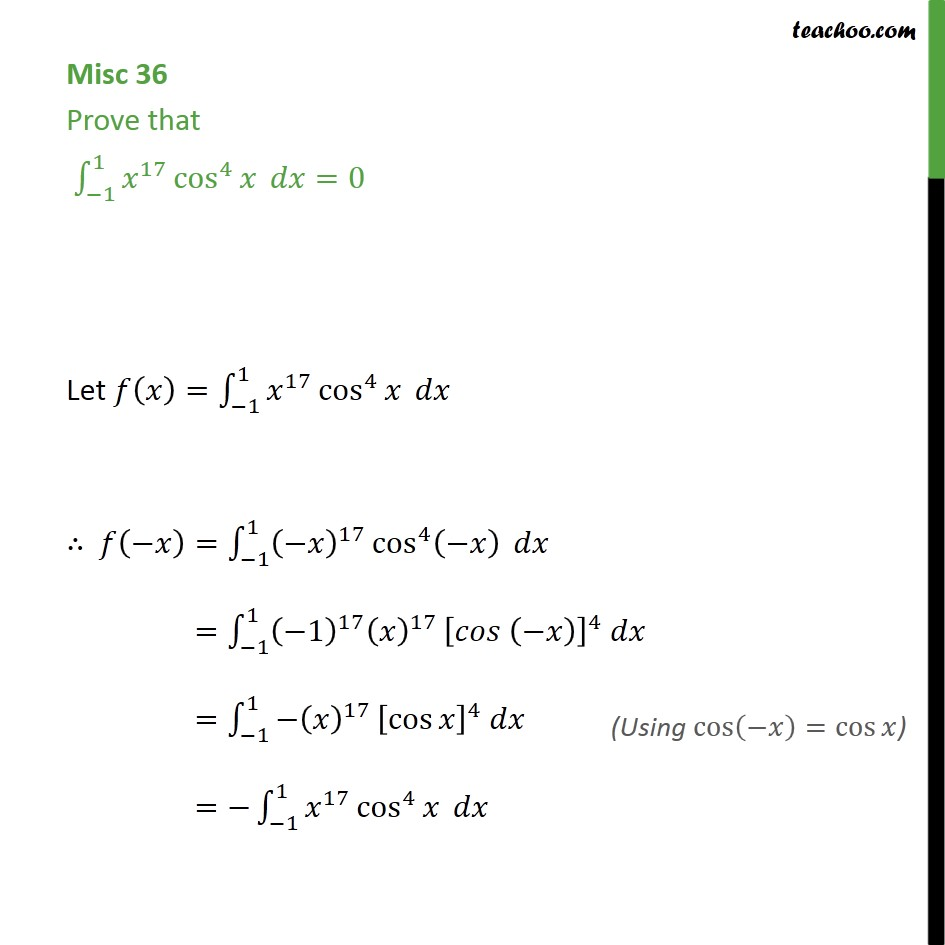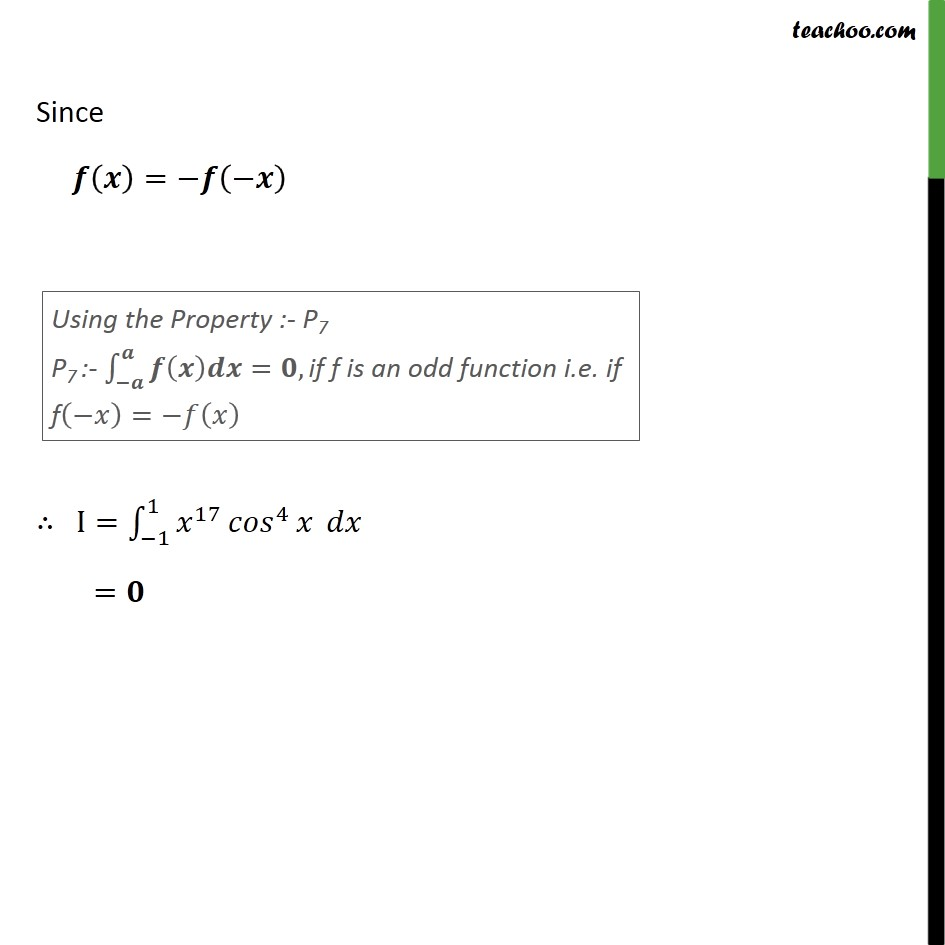Definite Integration by properties - P7

Chapter 7 Class 12 Integrals
Concept wiseIntroducing your new favourite teacher - Teachoo Black, at only ₹83 per month

### Transcript

Misc 36 Prove that 1 1 17 cos 4 =0 Let = 1 1 17 cos 4 = 1 1 17 cos 4 = 1 1 1 17 17 4 = 1 1 17 cos 4 = 1 1 17 cos 4 Since = I= 1 1 17 4 =